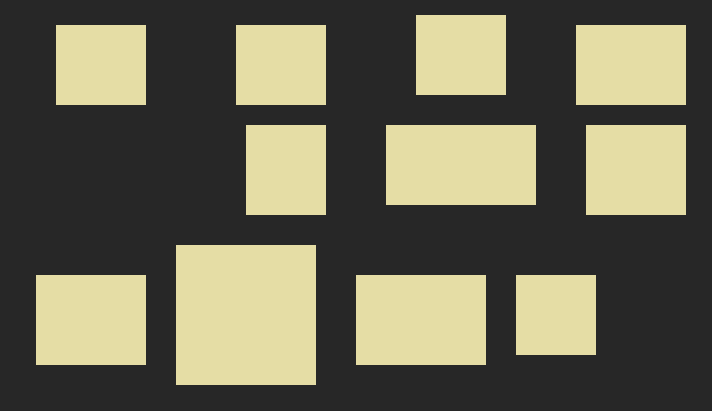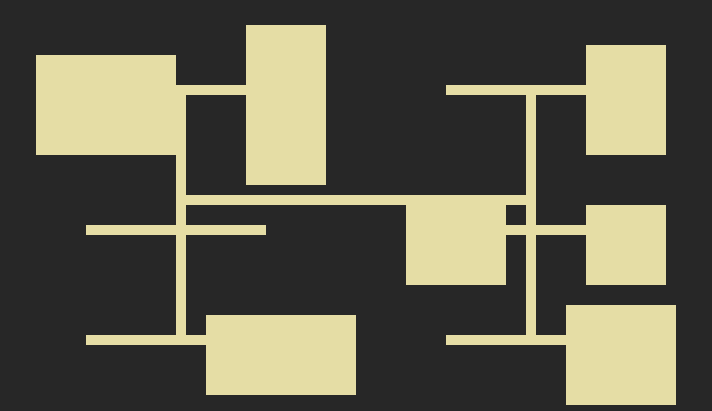# Procedural generation with Godot: Create dungeons using a BSP TreeA few months back we wrote an article showing how we created procedural caves in Godot using Cellular Automata.  Today we wanted to share a follow up, showing how to generate dungeons using a BSP Tree.

What is a BSP Tree?  I like this articles explanation, so rather than writing a bunch of paragraphs, I'll refer you to that article and just focus on the generation code below.sample dungeon generation using a bsp tree

### Getting Started

To follow along and see how this works you can download the project files from my github repo. The project is for Godot 3.2, and is just 1 Dungeon.tscn scene and about 200 lines of code.

To begin you want to create a scene in Godot and make the root node a TileMap, and then create a few tiles (you need ones for the ground and roof at least).  Next you want to attach a script to it.

As a first step, lets add some variables that will let us tweak the procedural generation.  Paste the following into your script you added to the TileMap:

``````extends TileMap

export(int) var map_w = 80
export(int) var map_h = 50
export(int) var min_room_size = 8
export(float, 0.2, 0.5) var min_room_factor = 0.4

enum Tiles { GROUND, TREE, WATER, ROOF }

var tree = {}
var leaves = []
var leaf_id = 0
var rooms = []``````

Once you save your script, if you select your TileMap node in the editor, you will now see some Script Variables show up:These variables show here because we used the export keyword, and will allow us to tweak how our dungeon is generated.

### Generation Code

``````func generate():
clear()
fill_roof()
start_tree()
create_leaf(0)
create_rooms()
join_rooms()

To generate our dungeon we first want to make a function describing all the tasks we need to do.  The first task, clear(), is a method of the TileMap node and simply clears it of any existing tiles.  The rest of the tasks we need to create.

First, we call fill_roof() to loop through all the cells we will use in our TileMap, defined by the map_w and map_h variables, and set them to be roof tiles:

``````func fill_roof():
for x in range(0, map_w):
for y in range(0, map_h):
set_cell(x, y, Tiles.ROOF)``````

Then we call start_tree() to start our actual BSP tree, with a root leaf that is the size of our map (padded in 1 cell).

``````func start_tree():
rooms = []
tree = {}
leaves = []
leaf_id = 0

tree[leaf_id] = { "x": 1, "y": 1, "w": map_w-2, "h": map_h-2 }
leaf_id += 1``````

Next is the meat of the whole generation, where we will recursively divide up our map into rectangles (leafs) and try and fit rooms in them, until there is no more space for rooms.

``````func create_leaf(parent_id):
var x = tree[parent_id].x
var y = tree[parent_id].y
var w = tree[parent_id].w
var h = tree[parent_id].h

# used to connect the leaves later
tree[parent_id].center = { x = floor(x + w/2), y = floor(y + h/2) }

# whether the tree has space for a split
var can_split = false

# randomly split horizontal or vertical
# if not enough width, split horizontal
# if not enough height, split vertical
var split_type = Util.choose(["h", "v"])
if   (min_room_factor * w < min_room_size):  split_type = "h"
elif (min_room_factor * h < min_room_size):  split_type = "v"

var leaf1 = {}
var leaf2 = {}

# try and split the current leaf,
# if the room will fit
if (split_type == "v"):
var room_size = min_room_factor * w
if (room_size >= min_room_size):
var w1 = Util.randi_range(room_size, (w - room_size))
var w2 = w - w1
leaf1 = { x = x, y = y, w = w1, h = h, split = 'v' }
leaf2 = { x = x+w1, y = y, w = w2, h = h, split = 'v' }
can_split = true
else:
var room_size = min_room_factor * h
if (room_size >= min_room_size):
var h1 = Util.randi_range(room_size, (h - room_size))
var h2 = h - h1
leaf1 = { x = x, y = y, w = w, h = h1, split = 'h' }
leaf2 = { x = x, y = y+h1, w = w, h = h2, split = 'h' }
can_split = true

# rooms fit, lets split
if (can_split):
leaf1.parent_id    = parent_id
tree[leaf_id]      = leaf1
tree[parent_id].l  = leaf_id
leaf_id += 1

leaf2.parent_id    = parent_id
tree[leaf_id]      = leaf2
tree[parent_id].r  = leaf_id
leaf_id += 1

# append these leaves as branches from the parent
leaves.append([tree[parent_id].l, tree[parent_id].r])

# try and create more leaves
create_leaf(tree[parent_id].l)
create_leaf(tree[parent_id].r)``````

Now that we have our map divided up into different sized rectangles using a BSP Tree, we want to create a room inside each leaf.  To make the map look less grid-like, we set a 75% chance of making the room, else its left blank.

``````func create_rooms():
for leaf_id in tree:
var leaf = tree[leaf_id]
if leaf.has("l"): continue # if node has children, don't build rooms

if Util.chance(75):
var room = {}
room.id = leaf_id;
room.w  = Util.randi_range(min_room_size, leaf.w) - 1
room.h  = Util.randi_range(min_room_size, leaf.h) - 1
room.x  = leaf.x + floor((leaf.w-room.w)/2) + 1
room.y  = leaf.y + floor((leaf.h-room.h)/2) + 1
room.split = leaf.split

room.center = Vector2()
room.center.x = floor(room.x + room.w/2)
room.center.y = floor(room.y + room.h/2)
rooms.append(room);

# draw the rooms on the tilemap
for i in range(rooms.size()):
var r = rooms[i]
for x in range(r.x, r.x + r.w):
for y in range(r.y, r.y + r.h):
set_cell(x, y, Tiles.GROUND)``````

At this point, you'll have your actual rooms show up on the map, which will look something like this:To make the map playable you need to connect the rooms.  To do this we go through each branch of the tree which gives us a sister pair of leaves.  We then connect these sister leaves using their center points.

``````func join_rooms():
for sister in leaves:
var a = sister
var b = sister
connect_leaves(tree[a], tree[b])

func connect_leaves(leaf1, leaf2):
var x = min(leaf1.center.x, leaf2.center.x)
var y = min(leaf1.center.y, leaf2.center.y)
var w = 1
var h = 1

# Vertical corridor
if (leaf1.split == 'h'):
x -= floor(w/2)+1
h = abs(leaf1.center.y - leaf2.center.y)
else:
# Horizontal corridor
y -= floor(h/2)+1
w = abs(leaf1.center.x - leaf2.center.x)

# Ensure within map
x = 0 if (x < 0) else x
y = 0 if (y < 0) else y

for i in range(x, x+w):
for j in range(y, y+h):
if(get_cell(i, j) == Tiles.ROOF): set_cell(i, j, Tiles.GROUND)``````

Some leafs will not contain rooms, either because they could not fit, or because we only set a 75% chance to make a room, so when we connect these leaves it will leave us with some dead end corridors.To clear these, we want to check every tile and see how many neighboring cells are roof cells.  If it has 3 roof cell neighbors, its a dead end so we clear it and try again, until there no more dead ends.

``````func clear_deadends():
var done = false

while !done:
done = true

for cell in get_used_cells():
if get_cellv(cell) != Tiles.GROUND: continue

var roof_count = check_nearby(cell.x, cell.y)
if roof_count == 3:
set_cellv(cell, Tiles.ROOF)
done = false

# check in 4 dirs to see how many tiles are roofs
func check_nearby(x, y):
var count = 0
if get_cell(x, y-1)   == Tiles.ROOF:  count += 1
if get_cell(x, y+1)   == Tiles.ROOF:  count += 1
if get_cell(x-1, y)   == Tiles.ROOF:  count += 1
if get_cell(x+1, y)   == Tiles.ROOF:  count += 1
return count``````

One final bit to mention, we used a Util class for a few methods to make things easier.  Here is that Util class and its methods:

``````extends Node

# Util.choose(["one", "two"])   returns one or two
func choose(choices):
randomize()

var rand_index = randi() % choices.size()
return choices[rand_index]

# the percent chance something happens
func chance(num):
randomize()

if randi() % 100 <= num: return true
else:                    return false

# returns random int between low and high
func randi_range(low, high):
return floor(rand_range(low, high))``````

You now have a simple dungeon generation working using a BSP Tree.  Just like we describe at the end of our cellular automata tutorial, you can make this a tool script in Godot so it can be run in the editor.